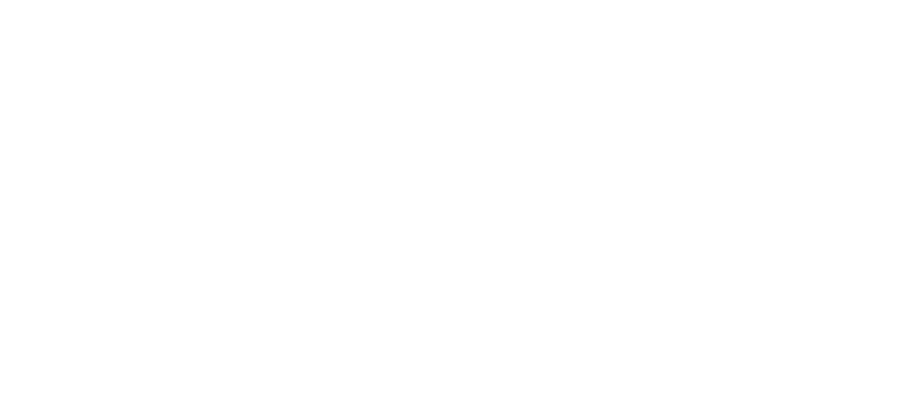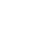# Power Electronics MCQ serires 1

Share

Home » Power Electronics MCQ serires 1

Top 20 questions of power electronics. For explanation of each answer you can watch my video

### Question 1: A thyristor is basically

• PNPN device
• A combination of diac and triac
• A set of SCRs
• A set of SCR, diac and a triac

### Question 2: A silicon controlled rectifier (SCR) is a

• Unijunction device
• Device with three junction
• Device with four junction
• None of the above

### Question 3: A thyristor equivalent of a thyratron tube is a

• Diac
• Triac
• Silicon controlled rectifier
• None of the above

### Question 4: An SCR is made up of silicon because

• Silicon has large leakage current than germanium
• Silicon has small leakage current than germanium
• Silicon has small leakage voltage than germanium
• Silicon has large leakage voltage than germanium

### Question 5: An SCR is considered to be a semi controlled device because

• It can be turned OFF but not ON with a gate pulse.
• It conducts only during one half cycle of an alternating current wave.
• It can be turned ON but not OFF with a gate pulse.
• It can be turned ON only during one half cycle of an AC.

### Question 6: Which statement is true for latching current ?

• It is related to turn off process of the device.
• It is related to conduction process of device.
• It is related to turn on process of the device.
• Both C and D.

• 0.01 A.
• 0.002 A.
• 0.009 A.
• 0.004 A

### Question 8: In reverse blocking mode of a thyristor

• junction J2 is in reverse bias and J1, J3 is in forward bias.
• junction J3 is in forward bias and J1, J2 in reverse bias.
• junction J1, J3 is in reverse bias and J2 is in forward bias.
• junction J1 and J2 is in forward bias and J3 is in reverse bias.

### Question 9: During the commutation period in 3 phase converter, overlap time is

• Dependent on the load current
• Dependent on the voltage
• Dependent on both the load current and load voltage behind the short circuit current

### Question 10: A UJT has ___________.

• Two pn junctions
• One pn junction
• Three pn junctions
• None of the above

### Question 11: The UJT may be used as ___________.

• Am amplifier
• A sawtooth generator
• A rectifier
• None of the above

### Question 12: The fig. below represents a

• Triac thyristor
• Diac trigger
• Diode rectifier
• None of the above

• One
• Two
• Three
• Four

### Question 14: What is a TRIAC?

• Two thyristors connected in series mode
• Two thyristors connected in parallel mode
• Two thyristors connected in anti parallel mode
• Two transistors connected in anti parallel mode

### Question 15: For an SCR, dv/dt protection is achieved through

• RC across SCR
• RL in series with SCR
• L across SCR
• L in series with SCR

### Question 16: Power diode is __________

• a three terminal semiconductor device
• a two terminal semiconductor device
• a four terminal semiconductor device
• a three terminal analog device

### Question 17: An ideal power diode must have

• low forward current carrying capacity
• large reverse breakdown voltage
• high ohmic junction resistance
• high reverse recovery time

### Question 18: A diode is said to be forward biased when the

• cathode is positive with respect to the anode
• anode is positive with respect to the cathode
• anode is negative with respect to the anode
• both cathode & anode are positive

### Question 19: To make a signal diode suitable for high current & high voltage carrying applications with minimum losses, ________

• a lightly doped n layer is grown between the two p & n layers
• a heavily doped n layer is grown between the two p & n layers
• a lightly doped p layer is grown between the two p & n layers
• a heavily doped p layer is grown between the two p & n layers

### Question 20: Which one of the following statements is TRUE for an ideal power diode?

• Reverse recovery time is non zero and reverse saturation current is zero
• Forward voltage drop is zero and reverse saturation current is non zero
• Forward voltage drop is non zero and reverse recovery time is zero
• Forward voltage drop is zero and reverse recovery time is zero

## FAQ

What is a thyristor ?

Thyristor is also called a silicon-controlled rectifier (SCR), it is basically a four-layer three-junction pnpn device. It has three terminals: anode, cathode, and gate.
Thyristor is basically an electronic switching device which can remain in conducting (on) and nonconducting state. However, it is a unidirectional device and can conduct only in one direction like a diode. The switching state of the device can be controlled by one of its terminals.

What is silicon controlled rectifier (SCR)?

The thyristor is also called a silicon-controlled rectifier (SCR), it is basically a four-layer three-junction pnpn device. It has three terminals: anode, cathode, and gate.
The silicon control rectifier (SCR) consists of four layers of semiconductors, which form NPNP or PNPN structures, having three P-N junctions labeled J1, J2 and J3, and three terminals.

Which thyristor is equivalent of a thyratron tube?

SCR, The thyratron is a type of gas-filled tube which is used as a high voltage electrical switch and a controlled rectifier.

Why SCR is considered to be a semi controlled device ?

During positive half cycle SCR is in forward blocking mode. By applying gate pulse the SCR can be turned ON during forward blocking mode. But SCR can not be turned OFF by applying gate pulse. It automatically turns off when anode current is below the Holding current. That is why it is called semi controlled rectifier.

What is latching current?

Latching current is the minimum required anode current to turn on SCR. Latching current is 2 or 3 times of holding current

What is holding current?

Holding current is the minimum anode current below which SCR get turn off. Holding current is 2 or 3 times less than latching current.

What is reverse blocking mode of a thyristor?

When a negative voltage is applied to the anode and a positive voltage to the cathode, the SCR is in reverse blocking mode, making J1 and J3 reverse biased and J2 forward biased.
The device behaves as two reverse-biased diodes connected in series. A small leakage current flows. This is the reverse blocking mode.

What is unijunction transistor (UJT)?

A unijunction transistor (UJT) is a three-lead electronic semiconductor device with only one junction that acts exclusively as an electrically controlled switch. The UJT is not used as a linear amplifier.

What is power diode?

It has two terminals anode and cathode same as that of a ordinary diode. In fact, a power diode is nothing but a signal diode with a extra layer.

Share

## One comment

1.Amod patel says: